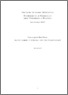# Alcune formule integrali nei Gruppi di Carnot

Montefalcone, F. (2005) Alcune formule integrali nei Gruppi di Carnot. Seminario di Analisi Matematica- Dipartimento di Matematica dell' Università di Bologna- Anno Accademico 2004/05 . pp. 1-21.

This is the most updated version of the document.

Full text available as:Preview PDF Download (363kB) | PreviewPreview PDF Download (348kB) | Preview

## Abstract

Let G a k-step Carnot group. In the 1st part of this talk we are concerned about Integral Geometry in the setting of Carnot groups. We start by illustrating an interplay between volume and H-perimeter, using one-dimensional horizontal slicing. This result is a kind of Fubini Theorem for H-regular hypersurfaces. Some applications are given: slicing of HBV-functions, integral geometric formulae for volume and H-perimeter and, making use of a suitable notion of convexity, we state a Cauchy type formula for this class of convex sets. We then state a sub-Riemannian Santalò formula showing some related applications: in particular we find two lower bounds for the 1st eigenvalue of the Dirichlet problem for the Carnot sub-Laplacian on smooth domains. In the second part we introduce some differential-geometric tools useful in the study of regular non-characteristic hypersurfaces. In particular, we state divergence-type theorems and integration by parts formulas with respect to the intrinsic H-perimeter measure on hypersurfaces. Finally we shall give a general formula for the 1st variation of the H-perimeter measure.

Abstract
Document type
Article
Creators
CreatorsAffiliationORCID
Montefalcone, F.
Keywords
Carnot Groups, Integral Geometry, Sub-Riemannian Geometry, BV spaces, H-perimeter, 1st variation
Subjects
DOI
Deposit date
04 Feb 2008